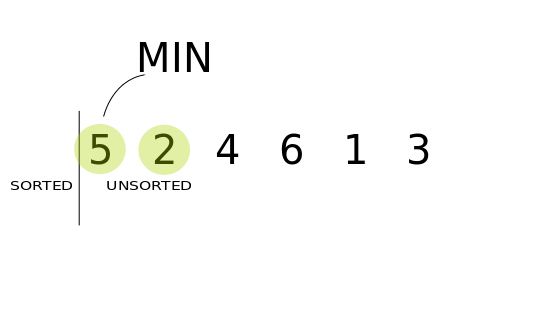#### 3 代码实现

```import java.util.Random;
​
public class SelectionSort{
//私有化构造方法
private SelectionSort(){};
//选择排序方法
public static void sort(int[] arr){
for(int i=0;i<arr.length-1;i++){
//这里，有一个比较巧妙的方法，就是for循环从数组的第0个元素开始，直到倒数第2个元素，每次都假定第i个元素是最小的元素，然后把这个最小元素的下标赋给一个变量来记录。
//每次循环的时候，都先假定这第i个元素是最小的，并把这个下标赋给minIndex.
int minIndex=i;
//接下来，就是要从第[i+1]开始到最后一个元素中，找出一个最小的元素，且该元素比minIndex还要小的那个元素。如果找到了的话，则把这2个元素交换位置。即：交换i和minIndex这2个元素。
for(int j = i+1;j < arr.length; j++){
if(arr[j] < arr[minIndex]){
//注意，这里找到的第j个元素不但比arr[minIndex]要小，而且它一定还是【i+1,arr.lengh】区间中最小的那个元素。如果找到了，则把我们原先假定的最小的那个元素，和这个真正的最小的元素交换位置。
minIndex = j;
}
}
swap(arr,i,minIndex);
}
}
//因为sort()是static的，所以这里的swap()也必须是static
private static void swap(int[] arr,int i;int j){
int temp=arr[i];
arr[i]=arr[j];
arr[j]=temp;
}

//写一个main方法，对排序算法进行测试
public static void main(String[] args){
int[] arr={7,2,5,4,3,100};
SelectionSort.sort(arr);
for(int key:arr){
System.out.print(key+"  ");
}
System.out.println();

Random random=new Random();
arr=new int;
for(int i=0;i<100;i++){
arr[i]=random.nextInt(1000);
}
SelectionSort.sort(arr);
for(int key:arr){
System.out.print(key+"  ");
}
}
}```

#### 4 “低效版”选择排序算法

```public class SelectionSort{
private SelectionSort(){};
public static void sortSlow(int[] arr){
for(int i=0;i<arr.length-1;i++){
for(int j=i+1;j<arr.length;j++){
if(arr[j]<arr[i]){
swap(arr,i,j);
}
}
}
}

private static void swap(int[] arr,int i,int j){
int temp=arr[i];
arr[i]=arr[j];
arr[j]=temp;
}
}```

#### 5选择排序算法和“低效版”选择排序算法性能测试对比

```import java.util.Arrays;
import java.util.Random;
​
/**
* @Author:asher
* @Date:2021/3/4 19:52
* @Description:DataStructureAlgorithm.SelectionSort
* @Version:1.0
*/
public class SelectionSort {
private SelectionSort(){}
​
public static void sort(int[] arr) {
for (int i = 0; i < arr.length - 1; i++) {
int minIndex = i;
for (int j = i+1; j <arr.length; j++) {
if (arr[j] < arr[minIndex]) {
minIndex = j;
}
}
swap(arr, i, minIndex);
}
}
​
public static void sortSlow(int[] arr) {
for (int i = 0; i < arr.length - 1; i++) {
for (int j = i+1; j <arr.length ; j++) {
if (arr[j] < arr[i]) {
swap(arr, i,j);
}
}
}
}
​
private static void swap(int[] arr, int i, int j) {
int temp = arr[i];
arr[i] = arr[j];
arr[j] = temp;
}
​
public static void main(String[] args) {
Random random = new Random();
int count=100000;
//初始化1个100000个元素的数组
int[] arr1 = new int[count];
for (int i = 0; i <count; i++) {
//实例化这个数组，每个元素都是随机生成，元素大小上限也为100000
arr1[i] = random.nextInt(count);
}
//copy这个数组，便于后续排序对比
int[] arr2 = Arrays.copyOf(arr1, arr1.length);

//选择排序算法测试
long t1=System.currentTimeMillis();
SelectionSort.sort(arr1);
long t2 = System.currentTimeMillis();
System.out.println("sort time ms:"+ (t2 - t1));
//"低效版"选择排序算法测试
long t3=System.currentTimeMillis();
SelectionSort.sortSlow(arr2);
long t4 = System.currentTimeMillis();
System.out.println("sortSlow time ms:"+ (t4 - t3));
}
}
​
//测试执行结果：
//sort time ms:4604
//sortSlow time ms:17710```

#### 6 带泛型的选择排序算法

```import java.util.Random;
public class SelectionSort{
private SelectionSort(){};
//这里只需要改为泛型方法即可，并不需要把类改为泛型类，因为该类被当做工具类来使用，并没涉及到带泛型的成员变量
//这里的参数中数组类型是带泛型T的，因此方法的返回值void前也需要指定泛型。
//同时T extends Comparable<T>,此处的extends意味着这个泛型类T是实现Comparable接口，而不是当做继承来理解
//此处要实现Comparable接口的原因是，arr[j]和arr[minIndex]此时被当做对象实例来比较，需要调用compareTo()方法，而不能再是使用小于<来比较大小了
public static <T extends Comparable<T>> void sort(T[] arr){
for(int i=0;i<arr.length-1;i++){
int minIndex=i;
for(int j=i+1;j<arr.length;j++){
//arr[j]和arr[minIndex]此时被当做对象实例来比较大小
if(arr[j].compareTo(arr[minIndex])<0){
minIndex=j;
}
}
swap(arr,i,minIndex);
}
}
​
private static <T> void swap(T[] arr,int i,int j){
T temp=arr[i];
arr[i]=arr[j];
arr[j]=temp;
}
​
public static void main(String[] args){
int count=100000;
Random random=new Random();
Integer[] arr=new Integer[count];
for(int i=0;i<count;i++){
arr[i]=random.nextInt(count);
}
long beginTime=System.currentTimeMillis();
SelectionSort.sort(arr);
long endTime=System.currentTimeMillis();
System.out.println("Generics Array selection sort time:"+(endTime-beginTime));
}
}
​
//输出结果：
//Generics Array selection sort time:13643```

#### 7 对自定义类使用选择排序进行排序

```public class Student implements Comparable<Student>{
private String name;
private int height;
private int age;

public Student(String name,int height,int age){
this.name=name;
this.height=height;
this.age=age;
}

@Override
public int compareTo(Student stu){
if(this.height!=stu.height){
return this.height-stu.height;
}else{
return this.age-stu.age;
}
}
@Override
public String toString(){
return "Student: name="+name+" height="+height+" age="+age;
}
}```

```public static void main(String[] args){
Student[] students={new Student("Jim",120,7),
new Student("Kate",120,7),
new Student("Tom",110,5)};
SelectionSort.sort(students);
for(Student stu:students){
System.out.println(stu);
}
}
//结果如下：
Student: name= Tom height=110 age=5
Student: name= kate height=120 age=7
Student: name= Jim height=120 age=7```

#### 8 时间复杂度分析

```public static <T extends Comparable<T>> void sort(T[] arr){
for(int i=0;i<arr.length-1;i++){
int minIndex=i;
for(int j=i+1;j<arr.length;j++){
//arr[j]和arr[minIndex]此时被当做对象实例来比较大小
if(arr[j].compareTo(arr[minIndex])<0){
minIndex=j;
}
}
swap(arr,i,minIndex);
}
}```

```SelectionSort ,10000 ,0.134 s
SelectionSort ,100000 ,13.731 s
-----------------------------------------------------------
10000 time: 0.082
100000 time: 12.608```